NCERT Questions

Class 10
Chapter 10 Class 10 - Light - Reflection and Refraction

## An object of size 7.0 cm is placed at 27 cm in front of a concave mirror of focal length 18 cm. At what distance from the mirror should a screen be placed, so that a sharp focussed image can be obtained? Find the size and the nature of the image.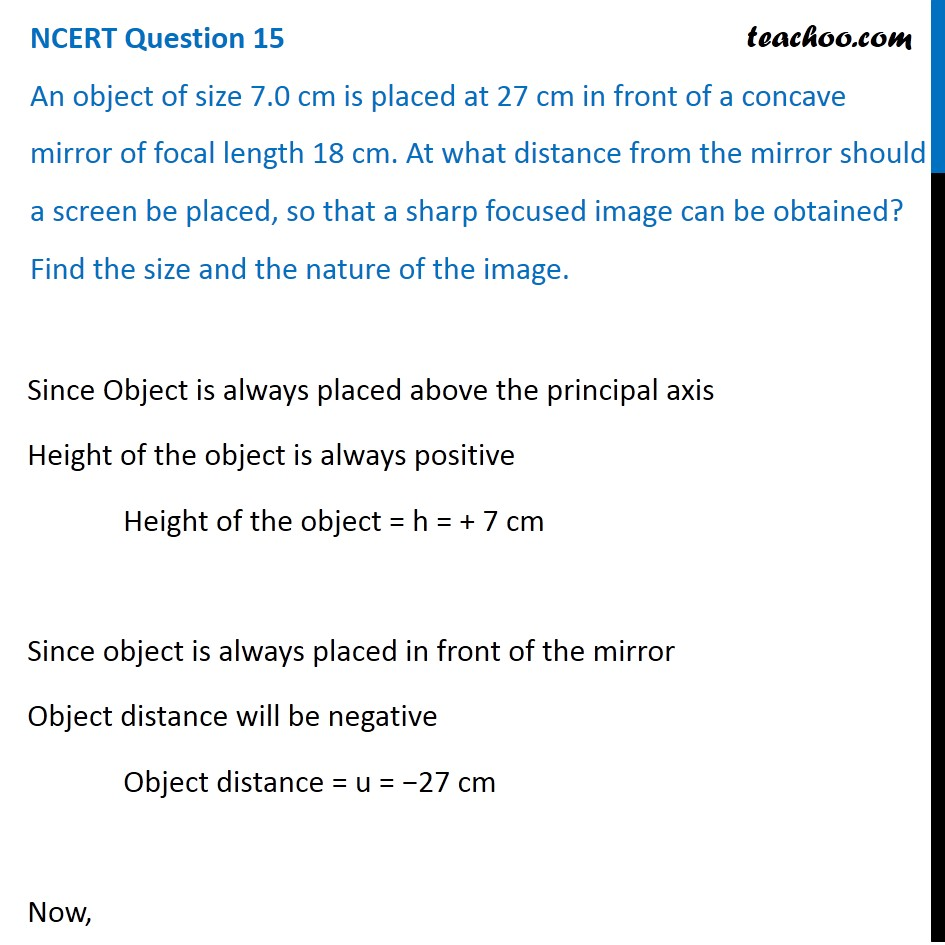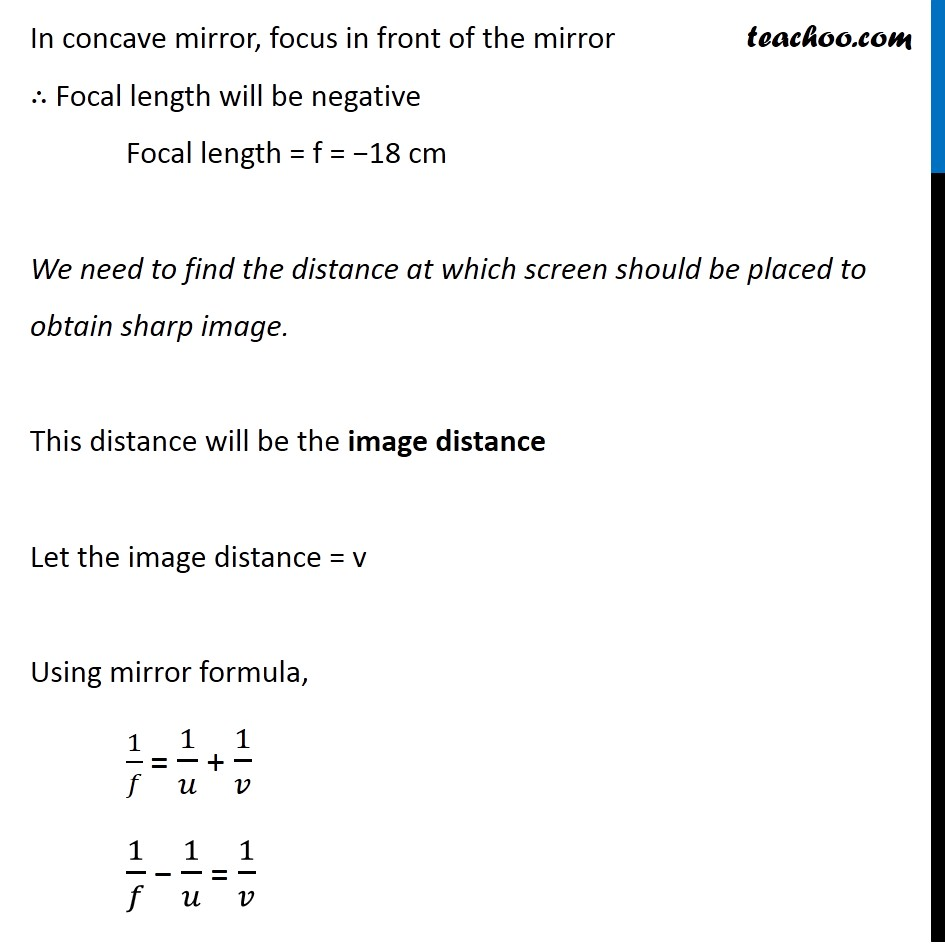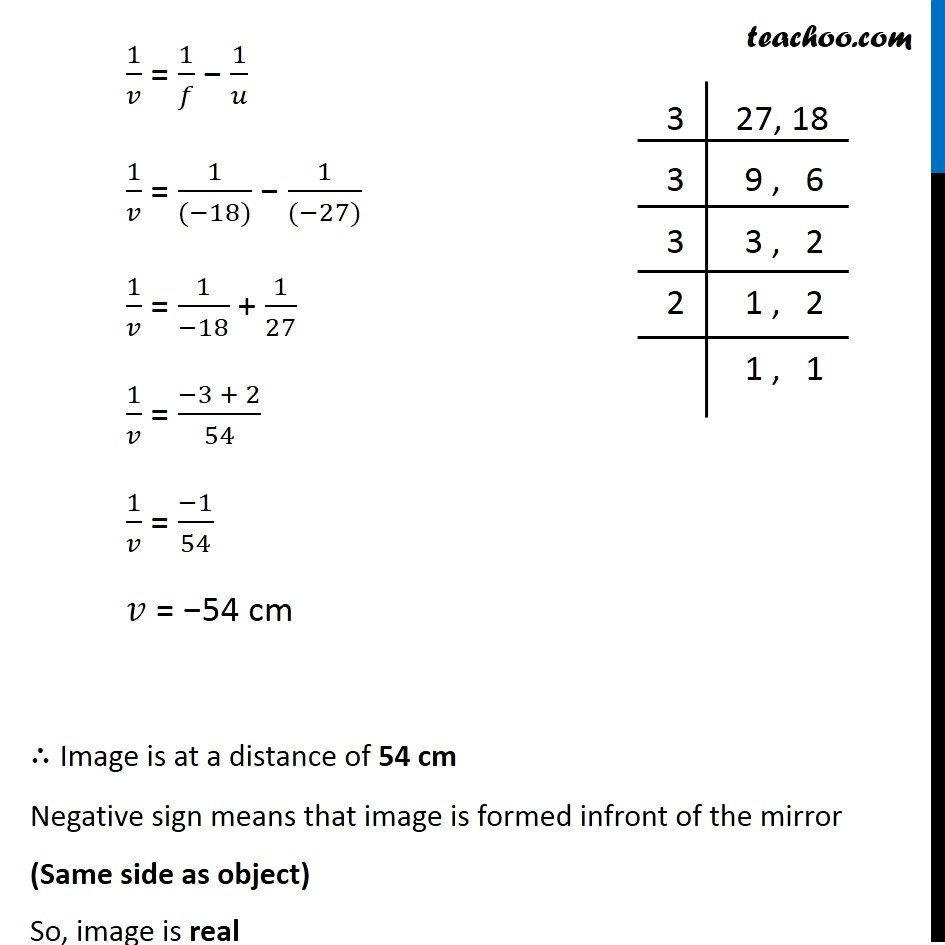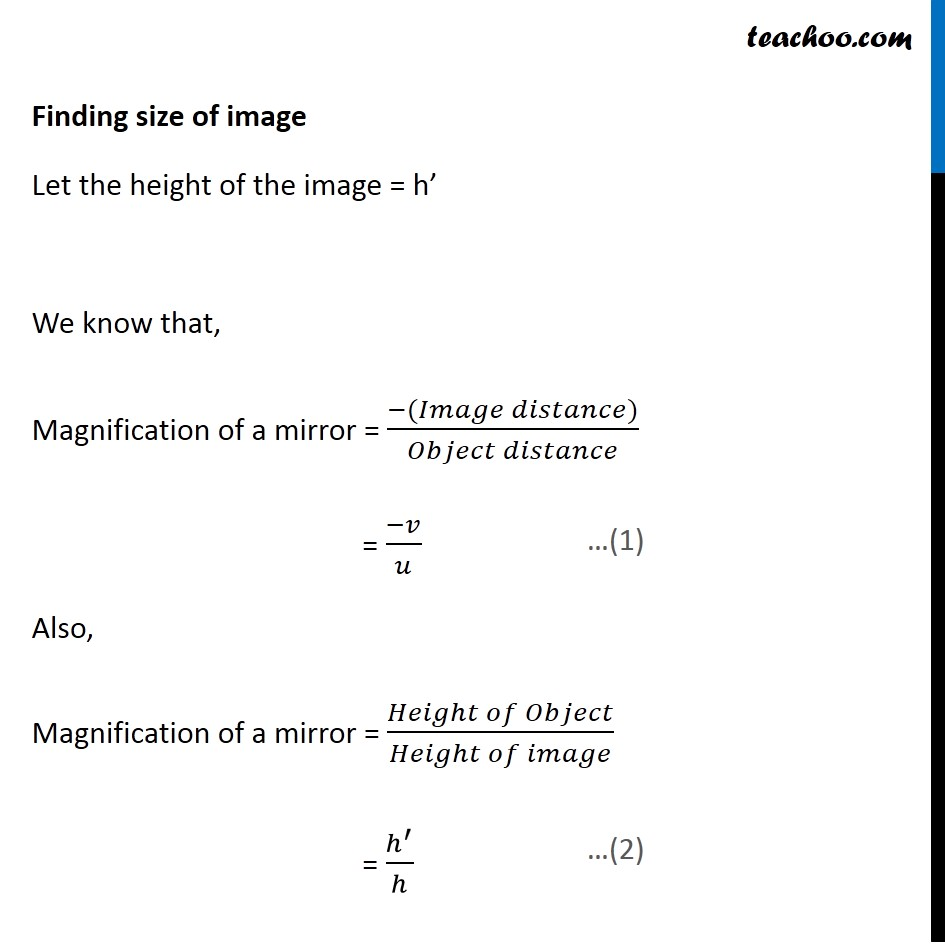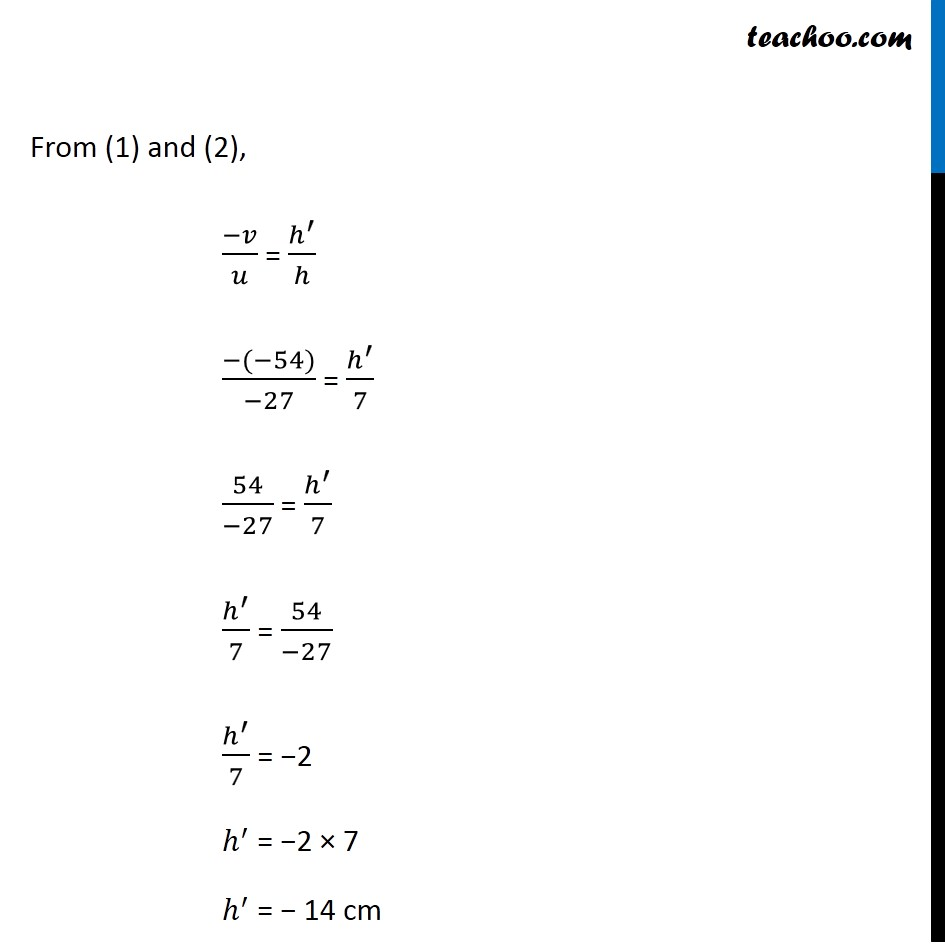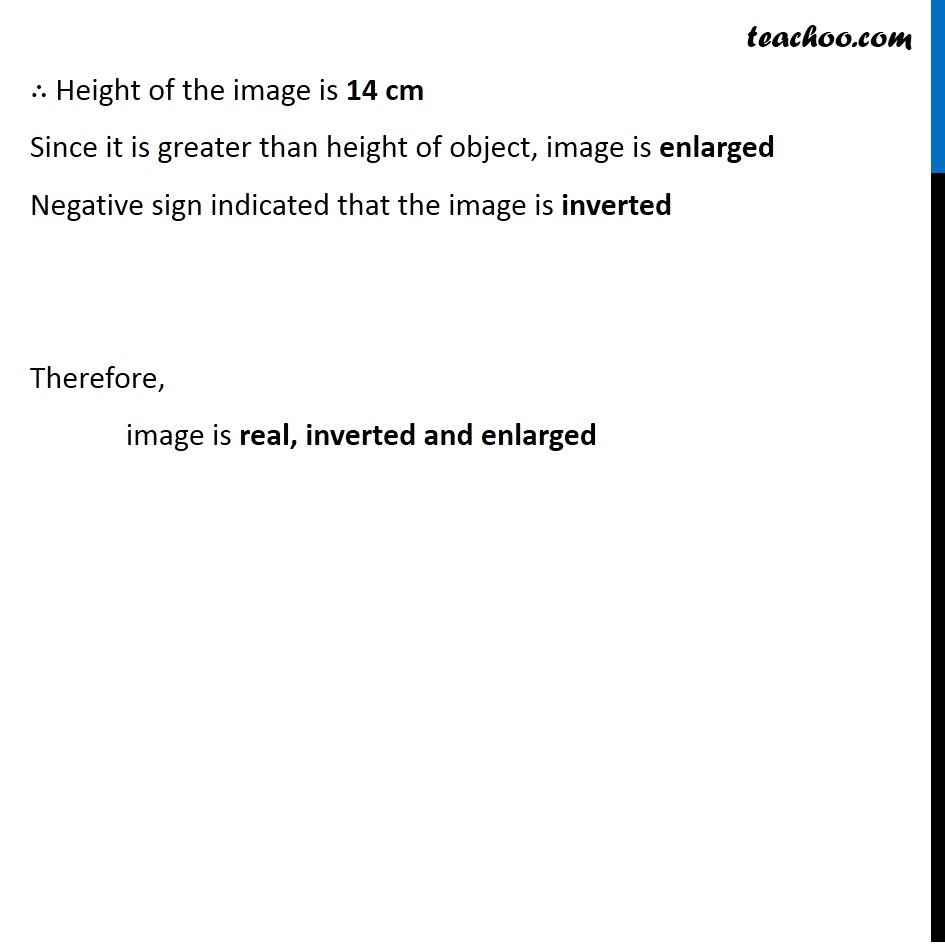Learn in your speed, with individual attention - Teachoo Maths 1-on-1 Class

### Transcript

NCERT Question 15 An object of size 7.0 cm is placed at 27 cm in front of a concave mirror of focal length 18 cm. At what distance from the mirror should a screen be placed, so that a sharp focused image can be obtained? Find the size and the nature of the image. Since Object is always placed above the principal axis Height of the object is always positive Height of the object = h = + 7 cm Since object is always placed in front of the mirror Object distance will be negative Object distance = u = −27 cm Now, In concave mirror, focus in front of the mirror ∴ Focal length will be negative Focal length = f = −18 cm We need to find the distance at which screen should be placed to obtain sharp image. This distance will be the image distance Let the image distance = v Using mirror formula, 1/𝑓 = 1/𝑢 + 1/𝑣 1/𝑓 − 1/𝑢 = 1/𝑣 1/𝑣 = 1/𝑓 − 1/𝑢 1/𝑣 = 1/((−18)) − 1/((−27)) 1/𝑣 = 1/(−18) + 1/27 1/𝑣 = (−3 + 2)/54 1/𝑣 = (−1)/54 𝑣 = −54 cm ∴ Image is at a distance of 54 cm Negative sign means that image is formed infront of the mirror (Same side as object) So, image is real Finding size of image Let the height of the image = h’ We know that, Magnification of a mirror = (−(𝐼𝑚𝑎𝑔𝑒 𝑑𝑖𝑠𝑡𝑎𝑛𝑐𝑒))/(𝑂𝑏𝑗𝑒𝑐𝑡 𝑑𝑖𝑠𝑡𝑎𝑛𝑐𝑒) = (−𝑣)/𝑢 Also, Magnification of a mirror = (𝐻𝑒𝑖𝑔ℎ𝑡 𝑜𝑓 𝑂𝑏𝑗𝑒𝑐𝑡)/(𝐻𝑒𝑖𝑔ℎ𝑡 𝑜𝑓 𝑖𝑚𝑎𝑔𝑒) = ℎ^′/ℎ From (1) and (2), (−𝑣)/𝑢 = ℎ^′/ℎ (−(−54))/(−27) = ℎ^′/7 54/(−27) = ℎ^′/7 ℎ^′/7 = 54/(−27) ℎ^′/7 = −2 ℎ^′ = −2 × 7 ℎ^′ = − 14 cm ∴ Height of the image is 14 cm Since it is greater than height of object, image is enlarged Negative sign indicated that the image is inverted Therefore, image is real, inverted and enlarged